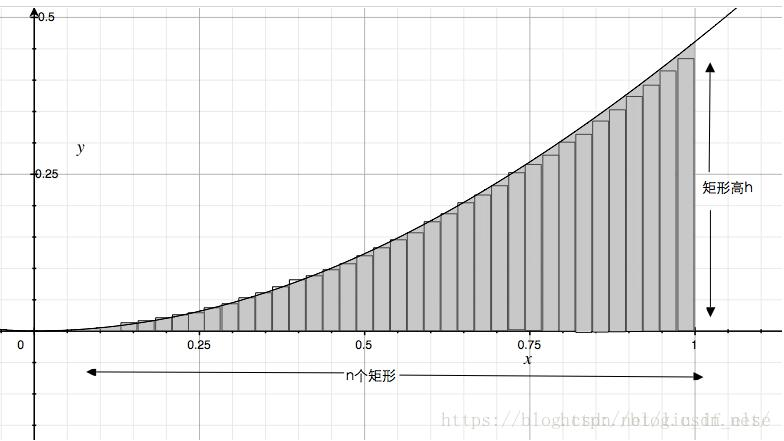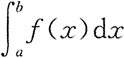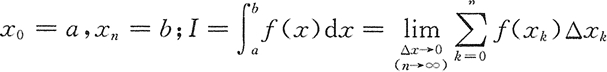• ## c语言实现定积分求解

万次阅读 多人点赞 2018-05-30 22:30:42
/*辛普生公式求定积分，公式为：S[n]=(4*T[2*n]-T[n])/3，其中T[2n],T[n]为梯形公式计算结果*/   double  Simposn( double  down,  double  up,  int  n)  {    double  s;      /*辛普生公式*/    ...
#include <stdio.h>  #include <stdlib.h>  #include <math.h>  #include <time.h>    #define N       3    double fun(double x)  {      double y;            y = sqrt(4-(x)*(x));      //y = sin(x);            return y;  }    /*随机点法求定积分*/  double Darts(int n)  {      double x, y;      time_t t;      int i = 0;      int count = 0;            srand((unsigned)time(&t));            for (i=0; i<n; i++)      {          x = rand()%100/100.0;          y = rand()%100/100.0;                    if (y <= 1-pow(x,2))          {              count++;              }         }            return (double)count/(double)n;  }    /*左矩形法求定积分*/  double LeftRect(double down, double up, int n)  {      double h, s;      int i;            /*计算步长*/      h = (up-down)/n;            s = fun(down)*h;            for (i=1; i<n; i++)      {          s = s + fun(down+i*h)*h;          }             return s;  }    /*梯形公式求定积分*/  double Trape(double down, double up, int n)  {      double h, s;      int i = 0;            /*计算步长*/      h = (up-down)/n;            s = 0.5*(fun(down)+fun(down+h))*h;            for (i=1; i<n; i++)      {          s = s + 0.5 * (fun(down+i*h) + fun(down+(i+1)*h))*h;      }            return s;     }     /*复合梯形公式*/  double T(double x, double y, int z)  {      double h, Tn;      int i = 0;            h = (y-x)/z;      Tn = (fun(x)+fun(y))/2;            for (i=0; i<z; i++)      {          Tn = Tn+fun(x+i*h);       }               Tn = Tn*h;            return Tn;  }     /*辛普生公式求定积分，公式为：S[n]=(4*T[2*n]-T[n])/3，其中T[2n],T[n]为梯形公式计算结果*/  double Simposn(double down, double up, int n)  {      double s;            /*辛普生公式*/      s = (4*T(down, up, 2*n) - T(down, up, n))/3;            return s;     }    /*高斯公式求定积分*/  double Gass(double (*func)(double x), double a, double b, int n)  {      int i = 0;            //高斯点及其求积系数列表      float x1={0.0};                                                        float A1={2};      float x2={-0.5573503,0.5573503};                                      float A2={1,1};      float x3={-0.7745967,0.0,0.7745967};                                   float A3={0.555556,0.888889,0.555556};      float x4={0.3399810,-0.3399810,0.8611363,-0.8611363};                  float A4={0.6521452,0.6521452,0.3478548,0.3478548};      float x5={0.0,0.5384693,-0.5384693,0.9061799,-0.9061799};             float A5={0.5688889,0.4786287,0.4786287,0.2369269,0.2369269};             float *p, *t;      switch (n)      {          case 1:                        p = x1;              t = A1;              break;          case 2:                        p = x2;              t = A2;              break;          case 3:                        p = x3;              t = A3;              break;          case 4:                        p = x4;              t = A4;              break;          case 5:                        p = x5;              t = A5;              break;          default :                 printf("intput wrong!");      }            float g = 0;      for (i=0; i<n; i++)      {          g += (*func)((b-a)*p[i]/2+(a+b)/2)*t[i];      }       g *= (b-a)/2;            return g;  }    int main(int argc, char *argv[])  {      printf("随机点法积分值%f\n", Darts(10000));            double down, up;      int n;      double sum = 0;            printf("积分下限:\n");      scanf("%lf", &down);      printf("积分上限:\n");      scanf("%lf", &up);      printf("分隔数目:\n");      scanf("%d", &n);            sum = LeftRect(down, up, n);      printf("左矩形法积分值为:%f\n", sum);            sum = Trape(down, up, n);      printf("梯形公式积分值为:%f\n", sum);            sum = Simposn(down, up, n);      printf("辛普生公式积分值为:%f\n", sum);            sum = Gass(fun, down, up, N);      printf("高斯公式积分值为:%f\n", sum);            return 0;  }
展开全文• 主要介绍了C语言实现定积分的方法,涉及C语言操作相关数学函数的技巧,具有一定参考借鉴价值,需要的朋友可以参考下
• 本文实例为大家分享了C语言程序实现黎曼和求定积分，供大家参考，具体内容如下通过黎曼和解定积分既是把在xy平面中函数曲线与x轴区间区域划分成多个矩形并求它们的面积之和，矩形数量越多，得出的面积越精确。...

本文实例为大家分享了C语言程序实现黎曼和求定积分，供大家参考，具体内容如下
通过黎曼和解定积分既是把在xy平面中函数曲线与x轴区间区域划分成多个矩形并求它们的面积之和，矩形数量越多，得出的面积越精确。
#include
#include
#include
#include
int main(){
float function1(float); //函数f(x)1
float function2(float); //函数f(x)2
float function3(float); //函数f(x)3
void integration(float f(float),float,float); //求定积分方法，参数为，函数fx，区间[a,b]的两个点
int result_a=integration(function1,1,0);
int result_b=integration(function2,1,-1);
int result_c=integration(function3,2,0);
}
void integration(float f(float),float endPos,float startPos) //求定积分方法，参数为，函数fx，区间[a,b]的两个点
{
float x;
float totalArea=0; //totalArea,所有矩形的总面积
float n=1000; //将函数曲线下方划为n个矩形，n值越大，精确值越高
float width; //单个矩形宽度
float area=0; //单个矩形面积
width=(endPos-startPos)/n; //求单个矩形宽度，既是函数总长度除以矩形数量
for(float i=1;i<=n;i++) //计算每个矩形的面积
{
x=startPos+width*i; //转入到xy平面， 通过i的递增，得出每个矩形底部x的值，以求矩形高度
area=f(x)*width; //用x做实参调用函数进一步求出y值，既矩形的高度，再用底乘高得出面积
totalArea=totalArea+area; //各个矩形面积相加
}
printf("the value of function is %f",t2);
}
float function1(float x){ //函数f(x)1
float y;
y=sin(x);
return y;
}
float function2(float x){ //函数f(x)2
float y;
y=cos(x);
return y;
}
float function3(float x){ //函数f(x)3
float y;
y=exp(x);
return y;
}以上就是本文的全部内容，希望对大家的学习有所帮助，也希望大家多多支持我们。
时间： 2020-02-26

展开全文• 主要为大家详细介绍了用C语言程序实现黎曼和求定积分，文中示例代码介绍的非常详细，具有一定的参考价值，感兴趣的小伙伴们可以参考一下
• ## c语言实现求定积分

千次阅读 2015-02-08 18:21:32
#include #include double dj(double Min,double Max,double flag=0.00001)//最大值，最小值，精度 { double i,j=0;
#include <cmath>
#include <cstdio>
double dj(double Min,double Max,double flags=0.00001)//最大值，最小值，精度
{
double i,j=0;
if(Min>Max)return 0;
for(i=Min;i<Max;i+=flags)
{
j+=sin(flags); //这里求的是sin的
}
return j;
}
int main()
{
printf("%.6lf",dj(0,3.1415926));
}

展开全文printf 数学
• 利用梯形法计算定积分 其中， f(x)=x3+3x2-x+2。 算法思想 根据定积分的定义分析可得：[x0,x1]，[x1,x2]，···，[xn-1,xn]，将定积分的区间 [a,b] 分成 n 个子区间，其中： 若右边的极限存在，其极限值即...
利用梯形法计算定积分其中， f(x)=x3+3x2-x+2。
算法思想
根据定积分的定义分析可得：[x0,x1]，[x1,x2]，···，[xn-1,xn]，将定积分的区间 [a,b] 分成 n 个子区间，其中：若右边的极限存在，其极限值即为定积分的值。理论上区间分得越细，越逼近定积分实际的值，一般采用梯形法近似计算定积分的值，把区间 [a,6] 划分成 n 等份，则任意第 f 个小梯形的面积为 (上底+下底)×高/2，si=H×[f(xi)-1)+f(xi)]/2，其中 xi+1=a+(i+1)×H；xi=a+i×H；H=(b-a)/n。该实例问题实际上转换为求 n 等份梯形的面积累计和。
程序
#include <stdio.h>
#include <math.h>
float collect(float s,float t,int m,float (*p)(float x));
float fun1(float x);
float fun2(float x);
float fun3(float x);
float fun4(float x);
int main()
{
int n,flag;
float a,b,v=0.0;
printf("Input the count range(from A to B)and the number of sections.\n");
scanf("%f%f%d",&a,&b,&n);
printf("Enter your choice：'1' for fun1,'2' for fun2,'3' for fun3,'4' for fun4==>");
scanf("%d",&flag);
if(flag==1)
v=collect(a,b,n,fun1);
else if(flag==2)
v=collect(a,b,n,fun2);
else if(flag==3)
v=collect(a,b,n,fun3);
else
v=collect(a,b,n,fun4);
printf("v=%f\n",v);
return 0;
}
float collect(float s,float t,int n,float (*p)(float x))
{
int i;
float f,h,x,y1,y2,area;
f=0.0;
h=(t-s)/n;
x=s;
y1=(*p)(x);
for(i=1;i<=n;i++)
{
x=x+h;
y2=(*p)(x);
area=(y1+y2)*h/2;
y1=y2;
f=f+area;
}
return (f);
}
float fun1(float x)
{
float fx;
fx=x*x-2.0*x+2.0;
return(fx);
}
float fun2(float x)
{
float fx;
fx=x*x*x+3.0*x*x-x+2.0;
return(fx);
}
float fun3 (float x)
{
float fx;
fx=x*sqrt(1+cos(2*x));
return(fx);
}
float fun4(float x)
{
float fx;
fx=1/(1.0+x*x);
return(fx);
}
调试运行结果
程序运行结果如下所示：
Input the count range(from A to B)and the number of sections.
0 1 100
Enter your choice：'1' for fun1,'2' for fun2,'3' for fun3,'4' for fun4==>2
v=2.750073
总结
① 定义 collect() 函数时，函数的首部 “float collect(float s,float t,int n,float (*p)(float x))” 中的 “float (*p)(float x)” 表示 p 是指向函数的指针变量，该函数的形参为实型。在 main() 函数的 if 条件结构中调用 collect() 函数时，除了将 a，b，n 作为实参传给 collect 的形参 s，n，t 外，还必须将函数名 fun1，fun2，fun3，fun4 作为实参将其入口地址传递给 collect() 函数中的形参 p。 ② 函数也是有地址的，函数名作为函数的首地址。可以定义一个指向函数的指针变量，将函数入口地址赋予指针变量，然后通过指针变量调用函数，这样的指针变量即称为指向函数的指针。 ③ 函数指针也是指针变量，可以实现指针变量的运算，但不能进行算术运算，因为函数指针的移动是毫无意义的，不同于数组指针变量，加减一个整数可以使指针指向后面或前面的数组元素。 ④ 在函数调用中 “(* 指针变量名)” 两边的括号不可少，其中此处为一种表示符号，而不是求值运算。
展开全文算法 数据结构 线性回归 推荐算法
• 本人想通过如下方式实现，但有个error 这种错误应该怎么消除
• ## 定积分的C语言实现

千次阅读 多人点赞 2018-09-24 15:41:04
想到求积分，我们第一步...这里我们就要回到定积分的最原始的定义，一切从定义出发就一定能算出来。 这篇我们先看一重的情况，对于二重积分，请参见https://blog.csdn.net/weixin_42612337/article/details/8289...
• 思路分析 代码实现 #include <stdio.h> float DefiniteIntegral(float x,float y,float (*f)(float)); float Fun(float x);... 功能描述：计算定积分的函数 形参列表：上限x 下限y 被积函数f 注意事c
• 用Romberg算法求下列积分，允许误差eps=0.00001 /***************************************************************** 《数值分析》实验习题2 机械工程 现代制造技术教育部重点实验室 ************************...数值分析 算法
• [C] 纯文本查看 复制代码#include #include void main( ){float f1(float x);float f2(float x);float f3(float x);float s1 = 0;float s2 = 0;float s3 = 0;... // 积分范围int i; // 循环变量prin...
• 本文实例为大家分享了C语言程序实现黎曼和求定积分，供大家参考，具体内容如下通过黎曼和解定积分既是把在xy平面中函数曲线与x轴区间区域划分成多个矩形并求它们的面积之和，矩形数量越多，得出的面积越精确。...
• int y)，并在主程序中实现输入输出4、编写计算学生年龄的递归函数5、编写递归函数实现Ackman函数一、实验目的与要求1、利用复化梯形公式计算定积分掌握C语言中定义函数的方法掌握通过“值传递”调用函数的方法2、...
• 复化辛普森Simpson求积公式 c语言实现 数值积分
• C语言定积分，对任意一个函数，本代码实现对其求定积分，可以改变积分上下限和步长
• C语言用六种方法求定积分C语言实验报告求定积分班级 10信息与计算科学一班姓名 戴良伟学号 2010750221描述问题利用①左矩形公式，②中矩形公式，③右矩形公式 ，④梯形公式，⑤simpson公式，⑥Gauss积分公式求解...
• 假设被积函数为   f x ，积分区间为   , a b ，把区间   ...根据定积分的定义及几 ...何意义，定积分就是求函数   f x 在区间   , a b 中图线下包围的...
• C语言__用六种方法求定积分C语言__用六种方法求定积分描述问题利用①左矩形公式，②中矩形公式，③右矩形公式 ，④梯形公式，⑤simpson公式，⑥Gauss积分公式求解定积分。分析问题2.1定积分21.1定积分的定义定积分...
• ## c语言实现积分运算

万次阅读 2018-10-24 21:24:44
用指针实现，最好先明白*p和（*p）的区别 int *p; //定义一个指针数组，该数组中每个元素是一个指针，每个指针指向哪里就需要程序中后续再定义了。 int (*p); //定义一个数组指针，该指针指向含4个元素...
• 通过黎曼和解定积分既是把在xy平面中函数曲线与x轴区间区域划分成多个矩形并求它们的面积之和，矩形数量越多，得出的面积越精确。 #include &lt;stdio.h&gt; #include &lt;string.h&gt; #include ...微积分 数学 编程
• 定积分的 sin (x)若要使用实用程序，用户必须输入两个值: b 和 c，在指定的范围 [b、 c]∫ 将计算 sin (x) dx。必须为弧度，不度输入 b 和 c请注意，对间隔的作用负积分也是否定的。例如，从 0 到 π 这个函数的积分......

# c语言实现定积分c语言 订阅